• ## 皇后问题

万次阅读 多人点赞 2018-11-29 15:44:55
皇后问题是一个以国际象棋为背景的问题：如何能够在 8×8 的国际象棋棋盘上放置八个皇后，使得任何一个皇后都无法直接吃掉其他的皇后？为了达到此目的，任两个皇后都不能处于同一条横行、纵行或斜线上。八皇后问题...
八皇后问题是一个以国际象棋为背景的问题：如何能够在 8×8 的国际象棋棋盘上放置八个皇后，使得任何一个皇后都无法直接吃掉其他的皇后？为了达到此目的，任两个皇后都不能处于同一条横行、纵行或斜线上。八皇后问题可以推广为更一般的n皇后摆放问题：这时棋盘的大小变为n1×n1，而皇后个数也变成n2。而且仅当 n2 ≥ 1 或 n1 ≥ 4 时问题有解。

皇后问题是非常著名的问题，作为一个棋盘类问题，毫无疑问，用暴力搜索的方法来做是一定可以得到正确答案的，但在有限的运行时间内，我们很难写出速度可以忍受的搜索，部分棋盘问题的最优解不是搜索，而是动态规划，某些棋盘问题也很适合作为状态压缩思想的解释例题。

进一步说，皇后问题可以用人工智能相关算法和遗传算法求解，可以用多线程技术缩短运行时间。本文不做讨论。

（本文不展开讲状态压缩，以后再说）

一般思路：

N*N的二维数组，在每一个位置进行尝试，在当前位置上判断是否满足放置皇后的条件（这一点的行、列、对角线上，没有皇后）。

优化1：

既然知道多个皇后不能在同一行，我们何必要在同一行的不同位置放多个来尝试呢？

我们生成一维数组record，record[i]表示第i行的皇后放在了第几列。对于每一行，确定当前record值即可，因为每行只能且必须放一个皇后，放了一个就无需继续尝试。那么对于当前的record[i]，查看record[0...i-1]的值，是否有j = record[k]（同列）、|record[k] - j| = | k-i |（同一斜线）的情况。由于我们的策略，无需检查行（每行只放一个）。

public class NQueens {
public static int num1(int n) {
if (n < 1) {
return 0;
}
int[] record = new int[n];
return process1(0, record, n);
}
public static int process1(int i, int[] record, int n) {
if (i == n) {
return 1;
}
int res = 0;
for (int j = 0; j < n; j++) {
if (isValid(record, i, j)) {
record[i] = j;
res += process1(i + 1, record, n);
}
}//对于当前行，依次尝试每列
return res;
}
//判断当前位置是否可以放置
public static boolean isValid(int[] record, int i, int j) {
for (int k = 0; k < i; k++) {
if (j == record[k] || Math.abs(record[k] - j) == Math.abs(i - k)) {
return false;
}
}
return true;
}
public static void main(String[] args) {
int n = 8;
System.out.println(num1(n));
}
}


优化2：

分析：棋子对后续过程的影响范围：本行、本列、左右斜线。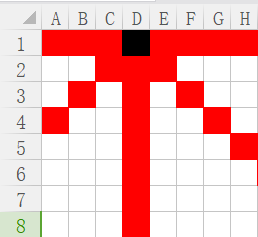黑色棋子影响区域为红色

本行影响不提，根据优化一已经避免

本列影响，一直影响D列，直到第一行在D放棋子的所有情况结束。

左斜线：每向下一行，实际上对当前行的影响区域就向左移动

比如：

尝试第二行时，黑色棋子影响的是我们的第三列；

尝试第三行时，黑色棋子影响的是我们的第二列；

尝试第四行时，黑色棋子影响的是我们的第一列；

尝试第五行及以后几行，黑色棋子对我们并无影响。

右斜线则相反：

随着行序号增加，影响的列序号也增加，直到影响的列序号大于8就不再影响。

我们对于之前棋子影响的区域，可以用二进制数字来表示，比如:

每一位，用01代表是否影响。

比如上图，对于第一行，就是00010000

尝试第二行时，数字变为00100000

第三行：01000000

第四行：10000000

对于右斜线的数字，同理：

第一行00010000，之后向右移：00001000，00000100，00000010，00000001，直到全0不影响。

同理，我们对于多行数据，也同样可以记录了

比如在第一行我们放在了第四列：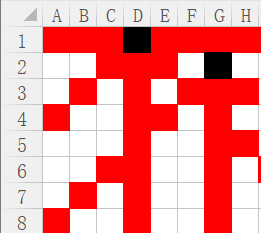第二行放在了G列，这时左斜线记录为00100000（第一个棋子的影响）+00000010（当前棋子的影响）=00100010。

到第三行数字继续左移：01000100，然后继续加上我们的选择，如此反复。

这样，我们对于当前位置的判断，其实可以通过左斜线变量、右斜线变量、列变量，按位或运算求出（每一位中，三个数有一个是1就不能再放）。

具体看代码：

注：怎么排版就炸了呢。。。贴一张图吧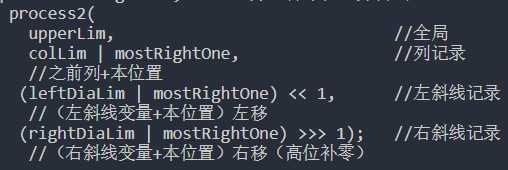public class NQueens {
public static int num2(int n) {
// 因为本方法中位运算的载体是int型变量，所以该方法只能算1~32皇后问题
// 如果想计算更多的皇后问题，需使用包含更多位的变量
if (n < 1 || n > 32) {
return 0;
}
int upperLim = n == 32 ? -1 : (1 << n) - 1;
//upperLim的作用为棋盘大小，比如8皇后为00000000 00000000 00000000 11111111
//32皇后为11111111 11111111 11111111 11111111
return process2(upperLim, 0, 0, 0);
}

public static int process2(int upperLim, int colLim, int leftDiaLim,
int rightDiaLim) {
if (colLim == upperLim) {
return 1;
}
int pos = 0;            //pos：所有的合法位置
int mostRightOne = 0;   //所有合法位置的最右位置

//所有记录按位或之后取反，并与全1按位与，得出所有合法位置
pos = upperLim & (~(colLim | leftDiaLim | rightDiaLim));
int res = 0;//计数
while (pos != 0) {
mostRightOne = pos & (~pos + 1);//取最右的合法位置
pos = pos - mostRightOne;       //去掉本位置并尝试
res += process2(
upperLim,                             //全局
colLim | mostRightOne,                //列记录
//之前列+本位置
(leftDiaLim | mostRightOne) << 1,      //左斜线记录
//（左斜线变量+本位置）左移
(rightDiaLim | mostRightOne) >>> 1);   //右斜线记录
//（右斜线变量+本位置）右移（高位补零）
}
return res;
}

public static void main(String[] args) {
int n = 8;
System.out.println(num2(n));
}
}


完整测试代码：

32皇后：结果/时间

暴力搜：时间就太长了，懒得测。。。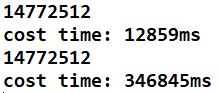public class NQueens {

public static int num1(int n) {
if (n < 1) {
return 0;
}
int[] record = new int[n];
return process1(0, record, n);
}

public static int process1(int i, int[] record, int n) {
if (i == n) {
return 1;
}
int res = 0;
for (int j = 0; j < n; j++) {
if (isValid(record, i, j)) {
record[i] = j;
res += process1(i + 1, record, n);
}
}
return res;
}

public static boolean isValid(int[] record, int i, int j) {
for (int k = 0; k < i; k++) {
if (j == record[k] || Math.abs(record[k] - j) == Math.abs(i - k)) {
return false;
}
}
return true;
}

public static int num2(int n) {
if (n < 1 || n > 32) {
return 0;
}
int upperLim = n == 32 ? -1 : (1 << n) - 1;
return process2(upperLim, 0, 0, 0);
}

public static int process2(int upperLim, int colLim, int leftDiaLim,
int rightDiaLim) {
if (colLim == upperLim) {
return 1;
}
int pos = 0;
int mostRightOne = 0;
pos = upperLim & (~(colLim | leftDiaLim | rightDiaLim));
int res = 0;
while (pos != 0) {
mostRightOne = pos & (~pos + 1);
pos = pos - mostRightOne;
res += process2(upperLim, colLim | mostRightOne,
(leftDiaLim | mostRightOne) << 1,
(rightDiaLim | mostRightOne) >>> 1);
}
return res;
}

public static void main(String[] args) {
int n = 32;

long start = System.currentTimeMillis();
System.out.println(num2(n));
long end = System.currentTimeMillis();
System.out.println("cost time: " + (end - start) + "ms");

start = System.currentTimeMillis();
System.out.println(num1(n));
end = System.currentTimeMillis();
System.out.println("cost time: " + (end - start) + "ms");
}
}



展开全文• ## n皇后问题-回溯法求解

万次阅读 多人点赞 2018-07-03 19:19:56
n皇后问题-回溯法求解 1.算法描述 在n×n格的国际象棋上摆放n个皇后，使其不能互相攻击，即任意两个皇后都不能处于同一行、同一列或同一斜线上，问有多少种摆法。 n皇后是由八皇后问题演变而来的。该问题是...
n皇后问题-回溯法求解
1.算法描述
在n×n格的国际象棋上摆放n个皇后，使其不能互相攻击，即任意两个皇后都不能处于同一行、同一列或同一斜线上，问有多少种摆法。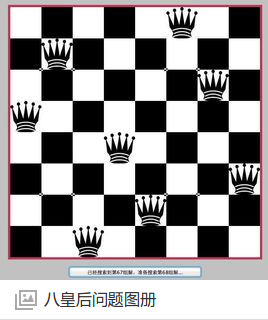n皇后是由八皇后问题演变而来的。该问题是国际西洋棋棋手马克斯·贝瑟尔于1848年提出：在8×8格的国际象棋上摆放八个皇后，使其不能互相攻击，即任意两个皇后都不能处于同一行、同一列或同一斜线上，问有多少种摆法。 高斯认为有76种方案。1854年在柏林的象棋杂志上不同的作者发表了40种不同的解，后来有人用图论的方法解出92种结果。
2.算法分析
随着计算机的普及和发展，以前人们无法解决的问题，计算机可以简单计算出来。而且思路十分清晰，那就是暴力求解，遍历所有情况，然后计算出解的个数。
2.1 回溯法
按选优条件向前搜索，以达到目标。但当探索到某一步时，发现原先选择并不优或达不到目标，就退回一步重新选择，这种走不通就退回再走的技术为回溯法
2.2 回溯法思路
用数组模拟棋盘，从第一行开始，依次选择位置， 如果当前位置满足条件，则向下选位置， 如果不满足条件，那么当前位置后移一位。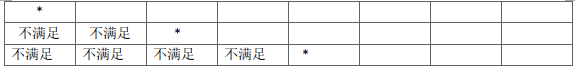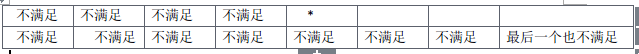最后一个不满足，回溯到上一行， 选下个位置，继续试探。
其实并不需要一个n*n的数组，我们只需要一个n长度的数组来存位置。
表示方式： arr[i] = k; 表示： 第i行的第k个位置放一个皇后。这样一个arr[n]的数组就可以表示一个可行解， 由于回溯，我们就可以求所有解。
2.3 n皇后回溯求解
因为八皇后不能在同行，同列， 同斜线。

每一行放一个皇后，就解决了不在同行的问题。
在第i行的时候，遍历n列，试探位置。和之前所有行放的位置进行比较。
比较列：当前列col 不等于 之前 所有列。 即col != arr[i].
比较斜线， 因为不再同一斜率为1或者-1的斜线。(row - i) / (col - arr[i]) != 1 或 -1
可以取巧用绝对值函数: abs(row-i) != abs(col-arr[i])

我们可以提取比较方法：
public boolean comp(int row, int col){   //当前行和列
for (int i = 0; i < row; i++)  //比较之前row -1 列。
{
if(col == arr[i] || abs(row-i) != abs(col-arr[i]))  //如果在同一列，同一斜线
return false;
}
return true;
}

比较函数写完后， 就只剩下回溯过程， 我们采用递归的方式回溯，比较好理解。
//当前层  row
for (int i = 0; i < n; i++){
if(comp(row, i)){
arr[row] = i;
//同样方式遍历下一层。 row + 1
}
}

2.4 时间复杂度
最坏的情况： 每一行有n种情况，有n行， 所以时间复杂度为O(n^n)。
但是由于回溯法会提前判断并抛弃一些情况，使得时间复杂度并没有想象中那么高。但是说是O(n!)也不太对，具体怎么算，暂时也不太清楚。（之前想当然了，此处有修正，多谢评论中的提醒。）
2.5 空间复杂度
因为只用了arr[n]的数组，也就是O(n).
3.代码实现
public class NQueen {
private int n;
private long count;
private int[] arr;
//    private int nn;

public NQueen(int n){
this.n = n;
//      nn = (1 << n) - 1;
count = 0;
arr = new int[n];
}

/**
* row col   i  arr[i]
* @param row
* @param col
* @return
*/
public boolean Check(int row, int col){
for(int i = 0; i < row; i++){
if(col == arr[i] || Math.abs(row - i) == Math.abs(col - arr[i])) //在同一列或者在同一斜线。一定不在同一行
return false;
}
return true;
}

public void FindNQueen(int k) {
if (k == n) {   //求出一种解， count+1
count++;
return;
}

for (int i = 0; i < n; i++) {
if (Check(k, i)) {   //检查是否满足条件
arr[k] = i;      //记录
FindNQueen(k + 1);   //递归查找
}
}

}

public static void main(String args[]){
NQueen nQueen = new NQueen(13);
nQueen.FindNQueen(0);
System.out.println(nQueen.count);
}

}


4. 拓展，位运算+回溯法实现
虽然计算机算的很快，但是上诉方法实在是太慢了， java就更慢了。如何网上就有大佬给出了位运算求解。精妙的不行。
4.1 算法思路
我们不再用数组来存储位置，而是用一个整数k，k一开始等于0. 不是普通的0.我们也不比较了，直接用两个整数l和r 记录在斜线在当前行不能走的位置。如果是n皇后， 那么用一个整数
nn =  1 << n 表示结束。
举个栗子吧： 8皇后问题。
初始化 那么我们就变成二进制的角度来看这些初始化的数据吧。
k = 00000000, l = 00000000, r = 00000000; nn = 11111111; (<- 8个0 8个1)

k: 每个位置i的0表示没有皇后，1表示在第i个位置放了一个皇后。
l: 0表示之前所有的列中放的皇后斜率为-1的线上没有涉及这个位置， 1 表示涉及到了，不能放皇后
r: 同l， 所有斜率为1的线涉及的位置。

l和r的实现：
比如k = 00110001.  我要在第4个为位置放一个皇后， 假设l和r都没有涉及这个位置。
那么这个位置x= 00001000.

k = (k & x) = 00111001.
l = (l & x) << 1.
r = (r & x) >> 1.

假设l = 00110001， r = 00100010.下一行，l表示斜率为-1不能放的位置， 那么第i+1行 l 中所有为1的数字都需要向左移动一位，r需要向右移动一位。 l & x 也就是加上当前选中的位置一起移动。
4.2代码实现
   //k  当前已走了多少个位置。   l 左斜线不能走的位置， r 右斜线不能走的位置。
public void FindNQueen(int k, int l, int r){
if(k == nn){
count++;
return;
}

int z = nn & (~(k | l | r));  //能走的位置， 和nn取并可以去掉前面多余的1
while(z != 0){
int index = z & (~z+1);   //最右边的一个1， 即要放皇后的位置。
z -= index;   //去掉这个位置。

FindNQueen(k | index, (l|index)<<1, (r|index)>>1);   //查找下一个。
}

}

4.3 算法分析

时间复杂度，应该同上分析一致，去掉但是少了检查的for循环，应该是O(n!)
空间复杂度，只用了几个变量， O(1).

5. 展望
其实还有其他方式和更快的方式求解，比如位运算+多线程， 还有号称时间复杂度为O(1)，利用数学公式的构造法求解。扶我起来，我要继续学。


展开全文位运算
• 皇后问题皇后问题皇后问题皇后问题皇后问题皇后问题皇后问题皇后问题皇后问题
• ## N皇后问题

万次阅读 多人点赞 2019-01-15 18:11:58
n 皇后问题研究的是如何将 n 个皇后放置在 n×n 的棋盘上，并且使皇后彼此之间不能相互攻击。 上图为 8 皇后问题的一种解法。 给定一个整数 n，返回所有不同的 n 皇后问题的解决方案。 每一种解法包含一个明确的 n ...
问题描述
n 皇后问题研究的是如何将 n 个皇后放置在 n×n 的棋盘上，并且使皇后彼此之间不能相互攻击。上图为 8 皇后问题的一种解法。
给定一个整数 n，返回所有不同的 n 皇后问题的解决方案。
每一种解法包含一个明确的 n 皇后问题的棋子放置方案，该方案中 ‘Q’ 和 ‘.’ 分别代表了皇后和空位。
示例:
输入: 4
输出: [
[".Q…",  // 解法 1
“…Q”,
“Q…”,
“…Q.”],
["…Q.",  // 解法 2
“Q…”,
“…Q”,
“.Q…”]
]
解释: 4 皇后问题存在两个不同的解法。
解题思路代码
代码思路
一行一行地摆放，在确定一行中的那个皇后应该摆在哪一列时，需要当前列是否合法，如果合法，则将皇后放置在当前位置，并进行递归，回溯。每行都摆满皇后时，则产生了一种解法，将所有解法收集并返回。
判断合法：当前将要摆放’Q’的位置和其他已摆放‘Q’的位置不能在同一列，且不能在同一条45度斜线或135度斜线上。这里判断是否在同一条斜线上可通过当前将要摆放’Q’的位置和其他已摆放‘Q’的位置横坐标之差和纵坐标之差的绝对值是否相等来判断
class Solution {
public List<List<String>> solveNQueens(int n) {
char[][] chs=new char[n][n];
for(int i=0;i<n;i++){
for(int j=0;j<n;j++){
chs[i][j]='.';
}
}
List<List<String>> res=new ArrayList<>();
backTracing(chs,0,n,res);
return res;
}
public void backTracing(char[][] chs,int row,int n,List<List<String>> res){
//每行都摆满皇后时，则产生了一种解法
if(row==n){
return;
}
//一行一行地摆放，在确定一行中的那个皇后应该摆在哪一列时，需要当前列是否合法。
//如果合法，则将皇后放置在当前位置，并进行递归，回溯。
for(int col=0;col<chs.length;col++){
if(isValid(chs,row,col)){
chs[row][col]='Q';
//递归
backTracing(chs,row+1,n,res);
//回溯
chs[row][col]='.';
}
}
}
public List<String> chsToList(char[][] chs){
List<String> list=new ArrayList<>();
for(int i=0;i<chs.length;i++){
}
return list;
}
//判断合法：当前将要摆放'Q'的位置和其他已摆放‘Q’的位置不能在同一列，且不能在同一条45度斜线或135度斜线上。
//这里判断是否在同一条斜线上可通过当前将要摆放'Q'的位置和其他已摆放‘Q’的位置横坐标之差和纵坐标之差的绝对值是否相等来判断。
public boolean isValid(char[][] chs,int x,int y){
for(int i=0;i<=x;i++){
for(int j=0;j<chs.length;j++){
if(chs[i][j]=='Q'&&(j==y||Math.abs(x-i)==Math.abs(y-j))){
return false;
}
}
}
return true;
}
}



展开全文• ## 八皇后问题

千次阅读 多人点赞 2018-11-17 13:14:48
皇后问题非递归实现 八皇后问题递归方式 问题分析 八皇后问题：高斯于1850年提出的问题。 在8*8的棋盘上放置八个皇后，任意两个皇后都不能处于同一行、列或者同一斜线上。 分析： 首先要解决的两个问题...
 目录

问题分析

过程模拟

问题回答

八皇后问题非递归实现

八皇后问题递归方式

问题分析

八皇后问题：高斯于1850年提出的问题。

在8*8的棋盘上放置八个皇后，任意两个皇后都不能处于同一行、列或者同一斜线上。

分析：

首先要解决的两个问题：

1. 如何表示棋盘？

2. 如何获取皇后的位置信息，进而判断是否相互攻击？

解决思想：

解题思想就是问题回溯法。

过程模拟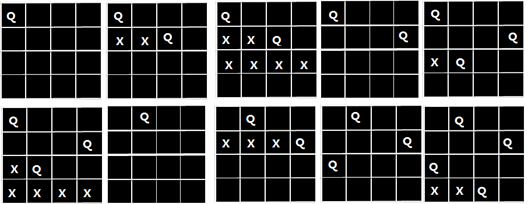当某个皇后探测到最后一列，而其后还有皇后没有摆放完全的时候，就需要回溯；

将本次探测皇后的上一个皇后向后再探测一个位置，继续下面的皇后的从0开始到最大列的探测；

如果回溯的过程中，直到第一个皇后到最大列位置，还不满足条件，也就是下次的回溯是-1（不存在）的时候，表示皇后的位置探测完毕；

如果在这个探测的过程中，没有输出满足条件的皇后位置序列，表示当前的棋盘和皇后序列不存在。

问题回答

Q1：

表示皇后，可以采用两种方式。

第一种是使用二维数组，用值来标识当前皇后在本位置存在。 如：int chess[N][N];

第二种是使用一位数组，用下标表示皇后的编号，用存放的值表示该皇后在棋盘中列的位置。如 int chess[N];

Q2:

假设皇后i和皇后j在棋盘上的位置是(i, Xi)，(j, Xj)；

不在同行：i≠j

不在同列：Xi≠Xj

不在同一条斜线上：|i-j|≠|Xi-Xj|  （也即是斜率的绝对值不等于1）

八皇后问题非递归实现

#include<iostream>
#include<cmath>
using namespace std;

const int N = 100;//假定最多能求的皇后问题
int x[N] = {-1};//这种方式下标表示皇后，值表示存放的列位置

//判断第k个皇后放置是否发生冲突
bool Success(int k){
//这里判断和前面的皇后是否发生冲突，所以前面皇后的下标是0-（k-1）
for(int i=0;i<k;i++){
//这里皇后的行for循环控制了的，所以可能行发生冲突
if(x[i]==x[k] || abs(k-i) == abs(x[i]-x[k]))
return false;//发生冲突
}
return true;//不发生冲突
}
//打印n皇后问题的一个解
void Print(int n){
for(int i=0;i<n;i++){//行
for(int j=0;j<n;j++){//列
if(j==x[i]){   //x[i]中存放的就是列下标
cout<<"Q  ";
}
else{
cout<<"0  ";
}
}
cout<<endl;
}
cout<<endl;
}
void Queue(int n){
int k=0, num=0; //num存放问题的解的个数
while(k>=0){//前面分析过，回溯到-1的时候，表示探测完毕
x[k]++;   //下一列摆放皇后k   , 初始k=-1,故而开始探测的起始位置列是0
//方式皇后后，就需要判断是否发生冲突
while(x[k]<n and !Success(k)){//发生冲突，就探测下一列
x[k]++;
}
//发生冲突会执行上面的，不发生冲突才执行下面
if(x[k]<n and k==n-1){ //k=n-1表示 最后一个皇后，x[k]<n表示位置有效
cout<<"第"<<++num<<"个解是："<<endl;
Print(n);
}
else if(x[k]<n and k<n-1){//表示还有皇后没有找到位置
//不发生冲突，还有皇后没放置完，故而需要放置下一个皇后
k++;
}else{
//探测越界 , 大于n-1, 表示本行没有可以放置的位置，所以回溯上一个元素
x[k--] = -1;  //回溯，并重新初始化上一个皇后所在的列下标
}
}
}

int main(void){
Queue(4);
return 0;
}


---------------------------------------------------分割线-------------------------------------------------

结果截图：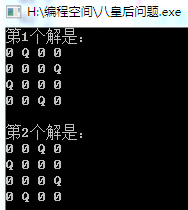八皇后问题递归方式

#include<iostream>
#include<cmath>
using namespace std;

const int N = 100;//假定最多能求的皇后问题
int chess[N][N]={0};
int n = 4;
bool Success(int k, int w){
for(int i=0;i<k;i++){//检查判断的始终都是前面的放置好位置的皇后
for(int j=0;j<n;j++){
if(chess[i][j]==1){
if(k==i or j==w or abs(i-k)==abs(j-w))
return false;
}
}
}
return true;
}
void Print(){
for(int i=0;i<n;i++){
for(int j=0;j<n;j++){
cout<<chess[i][j]<<" ";
}
cout<<endl;
}
cout<<endl;
}
int  num = 0;
void Queue(int row){
if(row==n){//假设n=8，八皇后row取值是0-7，故而在递归到row=8时就需要输出，
//然后退栈，再次row在调用的过程中row++,到row=8时输出，退栈，直到栈空
cout<<"第"<<++num<<"个八皇后序列输出："<<endl;
Print();
}else{
for(int j=0;j<n;j++){//模拟列
chess[row][j] = 1;
if(Success(row, j)){//如果成功，进行下一行的探测放置
Queue(row+1);
}
chess[row][j]=0;//撤回棋子
//递归调用，撤回棋子在最后一步，则如果探测下一个位置满足
//条件，直接进入下一个皇后的位置探测，不满足的时候，才会撤回
//棋子，同样的，在退栈的过程中，如果不满足，会回退到j++
//也就是上一个皇后进行下一个位置的探测,如此反复
}
}
}

int main(void){
Queue(0);
return 0;
}


-------------------------------------------------------分割线-------------------------------------------------

结果截图：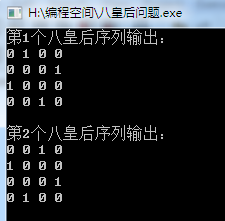附Java版本：

public List<List<String>> solveNQueens(int n) {
List<List<String>> result = new ArrayList<List<String>>();
boolean[][] matrix = new boolean[n][n];
backtrack(matrix, 0, result);
return result;
}

private void backtrack(boolean[][] matrix, int row, List<List<String>> result) {
int size = matrix.length;
if(row == size){
}else{
for (int i = 0; i < size; i++) {
if(!isValid(matrix, row, i)){
continue;
}
matrix[row][i] = true;
backtrack(matrix, row + 1, result);
matrix[row][i] = false;
}
}
}

private List<String> getResult(boolean[][] matrix) {
List<String> res = new ArrayList<String>();
for (boolean[] booleans : matrix) {
StringBuilder sb = new StringBuilder();
for (boolean aBoolean : booleans) {
sb.append(aBoolean ? 'Q' : '.');
}
}
return res;
}

private boolean isValid(boolean[][] matrix, int row, int k) {
for(int i=0;i<row;i++){//检查判断的始终都是前面的放置好位置的皇后
for(int j=0;j<matrix.length;j++){
if(matrix[i][j]){
if(j==k || Math.abs(i - row)== Math.abs(j - k))
return false;
}
}
}
return true;
}

作者：无涯明月

发文时间：2018-11-17

展开全文• 8皇后问题和由他推广得到的N皇后问题。题目来源于国际象棋的玩法，因为皇后所在的位置可以纵向、横向、两个斜向四个方向的“捕捉”，所以8皇后问题就是要求如何布置8个皇后在8*8的棋盘上而使他们互相无法“捕捉”。...
• 皇后问题，是一个古老而著名的问题，是回溯算法的典型案例。该问题是国际西洋棋棋手马克斯·贝瑟尔于1848年提出：在8×8格的国际象棋上摆放八个皇后，使其不能互相攻击，即任意两个皇后都不能处于同一行、同一列或...递归 C++
•递归
• N皇后问题 N皇后问题 经典的八皇后问题是在8×8格的国际象棋上摆放八个皇后，使其不能互相攻击，即任意两个皇后都不能处于同一行、同一列或同一斜线上，问有多少种摆法。现对该问题进行改良要求在一个n×n的棋盘上...c++ python 算法
• 终于搞定了用递归（dfs）来写n皇后和2n皇后问题，赶紧来总结一下，省的忘了= - = 什么是n皇后问题 首先要从8皇后问题说起 八皇后问题，是一个古老而著名的问题，是回溯算法的典型案例。该问题是国际西洋棋棋手...
• n皇后问题 　八皇后问题是一个以国际象棋为背景的问题：如何能够在 8×8 的国际象棋棋盘上放置八个皇后，使得任何一个皇后都无法直接吃掉其他的皇后？为了达到此目的，任两个皇后都不能处于同一条横行、纵行或斜线...
• n 皇后问题研究的是如何将 n 个皇后放置在 n×n 的棋盘上，并且使皇后彼此之间不能相互攻击。 给定一个整数 n，返回所有不同的 n 皇后问题的解决方案。 每一种解法包含一个明确的 n 皇后问题的棋子放置方案，该...算法
• 皇后问题是大数学家高斯于1850年提出来的。该问题是在8×8的国际象棋棋盘上放置8个皇后，使得没有一个皇后能“吃掉”任何其他一个皇后，即没有任何两个皇后被放置在棋盘的同一行、同一列或同一斜线上。 输出8皇后...
• 身为一只大二狗,表示很多东西都不会,包括n皇后问题之前理解的也不是很透彻,很羞愧的写下了这篇blog= = n皇后问题( hdu-2553) 在N*N的方格棋盘放置了N个皇后，使得它们不相互攻击（即任意2个皇后不允许处在同一排，...
• 【八皇后问题】就是回溯算法的典型，这篇博客给出了详细的实现逻辑以及完整的代码实现。递归算法...